GBP £

My bag - 0 Item(s)

Total:

£0.00

• Yamaha Serial Numbers will indicate the year of production. Below is a basic serial number guide.

>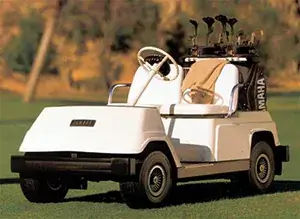Yamaha G1 Golf Cart began production in 1979.  The serial number is located near the drivers side rear tire underneath the golf cart.

G1 - GAS           G1 - ELECTRIC

J10-000101 = 1979           J14-000101 = 1980

J10-007705 = 1980           J14-100101 = 1981

J10-030101 = 1981           J22-000101 = 1982

J17-000101 = 1982           J26-000101 = 1983

J24-000101 = 1983           J36-000101 = 1984

J31-000101 = 1984           J36-010101 = 1985

J31-100101 = 1985           J36-011212 = 1986

J31-140101 = 1986           J31-159082 = 1989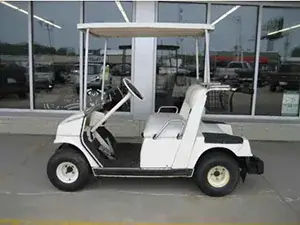The Yamaha G2 began production in 1985 and the serial number can be located in one of two places.  Either under the seat towards the front of the golf cart, or under the front bumper on the frame of the golf cart.

G2 - GAS           G2 - ELECTRIC

J38-000101 = 1985           J41-000101 = 1985

J38-007101 = 1986           J41-016101 = 1986

J38-020101 = 1987           J41-030101 = 1987

J38-071101 = 1988           J41-053101 = 1988

JA2-000001 = 1988           JE2-000001 = 1988

J55-000101 = 1989           J56-000101 = 1989

J55-100120 = 1990           J56-100101 = 1990

J55-200101 = 1991           J56-200101 = 1991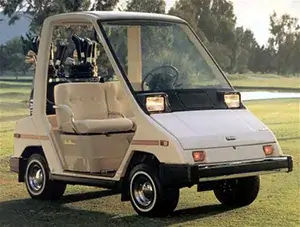The serial number on a Yamaha G3 Golf Cart is located near the passenger side rear tire underneath the golf cart.

G3 - GAS           G3 - ELECTRIC

J42-000101 = 1986           J46-000101 = 1987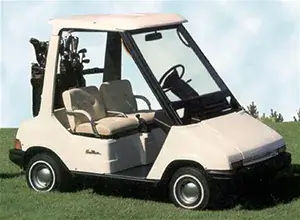The Yamaha G5 Golf Cart was introduced in 1990.  The serial number can be located underneath the front bumper area on the frame.

G5 - GAS           G5 - ELECTRIC

J50-000101 = 1990           J51-000101 = 1990

J50-100101 = 1991           J51-100101 = 1991

J50-200103 = 1992           J51-200101 = 1992

J50-300101 = 1993           J50-400101 = 1994

J50-500101 = 1995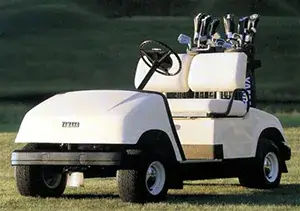The Yamaha G8 Golf Cart serial number is located under the passenger side seat towards the front of the golf cart.

G8 - GAS           G8 - ELECTRIC

JF2-000007 = 1990           JF3-000005 = 1990

JF2-100101 = 1991           JF3-100101 = 1991

JF2-200101 = 1992           JF3-200101 = 1992

JF2-300101 = 1993           JF3-300101 = 1993

JF2-400101 = 1994           JF3-400101 = 1994

JF3-401801 = 1994           JF3-402000 = 1995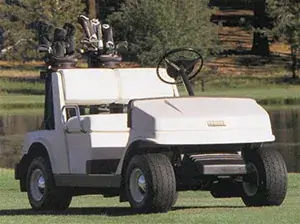The Yamaha G9 serial number location is underneath the seat towards the front of the golf cart.

G9 - GAS          G9 - ELECTRIC

JG5-000101 = 1991          JG6-000001 = 1991

JG5-100101 = 1992          JG6-100101 = 1992

JG5-200103 = 1993          JH7-000125 = 1992

JG5-300101 = 1994          JH7-100101 = 1993

JG5-400101 = 1995          JH7-200149 = 1994

JH7-201801 = 1994          JH7-300101 = 1995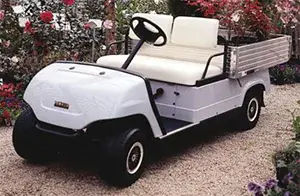The Yamaha G11 Serial Number location is underneath the seat towards the rear of the golf cart.

G11 - GAS

JJ3-000101 = 1993

JJ3-100101 = 1994

JJ3-200101 = 1996

JR3-000101 = 1997

JR3-100101 = 1998

JR3-200101 = 1999

JR3-300101 = 2000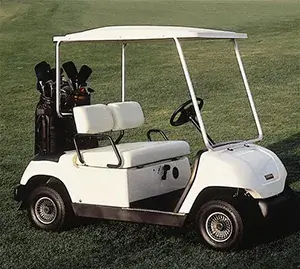The Yamaha G14 & G16 serial numbers are located underneath the drivers side seat towards the front of the golf cart.  Some models may have the serial number located inside the drivers side glove compartment as well.

G14 - GAS          G14 - ELECTRIC

JN3-100101 = 1995          JN4-100101 = 1995

JN3-200101 = 1996          JN4-200101 = 1996

G16 - GAS          G16 - ELECTRIC

JN6-000101 = 1996          JN8-000101 = 1996

JN6-100101 = 1997          JN8-100101 = 1997

JN6-200101 = 1998          JN8-200101 = 1998

JN6-300101 = 1999          JN8-300101 = 1999

JN6-400101 = 2000          JN8-400101 = 2000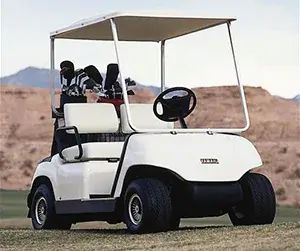The Yamaha G19 serial number is located inside the drivers side glove compartment or under the rear bumper attached to the frame.

G20 - GAS           G19 - ELECTRIC

JR8-000101 = 2000           JR1-000101 = 1996

JR8-100101 = 2001           JR1-100101 = 1997

JR8-200101 = 2002           JR1-200101 = 1998

G21 - GAS           JR1-300101 = 1999

JR6-000101 = 2001           JR1-400101 = 2000

JR6-100101 = 2002           JR1- 500101 = 2001

JR6-200101 = 2003           JRI-600101 = 2002

JR6-300101 = 2004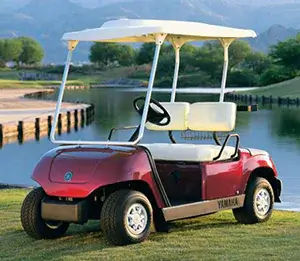The Yamaha G22 or better known as the G-MAX began production in 2003 and the serial number is located inside the drivers side glove compartment.

G22 - GAS (G-MAX)           G22 - ELECTRIC (G-MAX)

JU0-000101 = 2003          JU2-000101 = 2003

JU0-100101 = 2004          JU2-100101 = 2004

JU0-200101 = 2005          JU2-200101 = 2005

JU0-209201 = 2005          JU2-205701 = 2005

JU0-300101 = 2006          JU2-300101 = 2006

JU0-310101 = 2006          JU2-306201 = 2006

JU0-400101 = 2007          JU2-400101 = 2007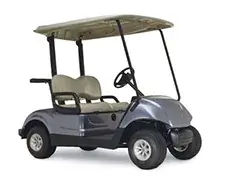The Yamaha Drive is the newest addition the the Yamaha Golf Car line and began production in 2007.  Thier serial number is located on the passenger side near the seat from 2007-2010.  In 2011 and up the serial number is located underneath the seat towards the rear of the golf cart.

YDR - GAS (DRIVE)          YDR - ELECTRIC (DRIVE)

JW1-000101 = 2007         JW2-000101 = 2007

JW1-100101 = 2008         JW2-100101 = 2008

JW1-200101 = 2009         JW2-200101 = 2009

JW1-300101 = 2010         JW2-300101 = 2010

JW8-000101 = 2011         JW9-000101 = 2011

JW8-100101 = 2012         JW9-100101 = 2012

JW8-200101 = 2012.5      JW9-200101 = 2012.5

JW8-300101 = 2013         JW9-300101 = 2013